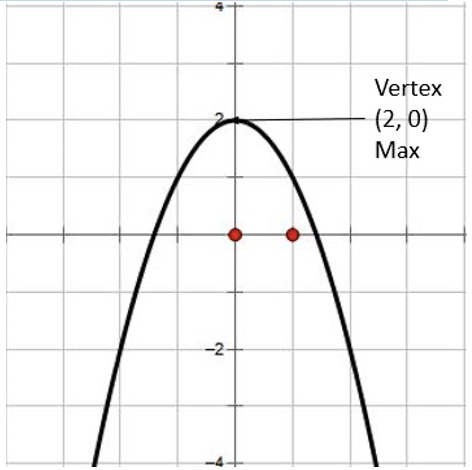# How to find the Vertex of a Parabola

The vertex of a parabola is the point of intersection of the parabola and the axis of symmetry. This can either be the lowest (minimum) or highest (maximum) point of the parabola. You can determine whether you have a minimum or maximum by either looking at its graph or by the equation itself.For identifying the minimum or maximum on a graph, look at the images below:

To determine if you have a maximum or a minimum from an equation, you will need to look at the value of the x<sup>2</sup>.

If POSITIVE, then your graph will be facing upwards and you will have a minimum.

If NEGATIVE, then your graph will be facing downwards and you will have a maximum.

## Vertex of a Parabola Problem Example 1:

Determine if y = -3<sup>2</sup> - 5x + 2, will have a maximum or a minimum.

The value with the x<sup>2</sup> is -3. Since this value is negative, the graph will be facing downwards and have a maximum.

## How do I find the Vertex of the Parabolafrom General Form?

Recall, that the General Form of a parabola is y = ax<sup>2</sup> + bx + c. In order to find the vertex from this form, you must first find the x-coordinate of the vertex which is x = - b/2a. After you find the x-coordinate of the vertex, you will take this number and substitute for x  in the parabola equation. You then will be able to find the y-coordinate of the vertex.

## Vertex of a Parabola Problem Example 2:

Find the vertex of the parabola y = x<sup>2</sup> - 6x + 3.

Step 1: Using the general form y = ax<sup>2</sup>  + bx + c, identify a and b.

a = 1 and b -6

Step 2: Substitute your values into x-coordinate formula and simplify.

Step 3: Substitute x = 3 into the equation y = x<sup>2</sup> - 6x + 3 and simplify.

y = x<sup>2</sup> - 6x + 3 = (3)<sup>2</sup> - 6(3) + 3 = 9 - 18 + 3 = -6

Thus, the vertex of the parabola is (3, -6)

### How do I find the Vertex of the Parabola from Standard Form?

Recall, that theStandard Form of a Parabola is y = a(x-h)<sup>2</sup> + k. To find the vertex in this form, you will use identify h and k from the standard form and put it into the vertex point (h, k).

## Vertex of a Parabola Problem Example 3:

Find the vertex of the parabola y = 2(x-1)<sup>2</sup> + 3.

Step 1: Using the Standard Form y = a(x - h)<sup>2</sup> + k, identify (h, k)

h = 1 and k = 3

The vertex is (1, 3).

#### MORE MATH PROBLEMS# Need more help? Get Algebra or Geometry Tutoring

Remind Math Tutoring provides 1 on 1 online math tutoring. Your experienced tutor can help you work through difficult problems and help you master all algebra concepts. How do you find the vertex of a parabola? No problem. The first session is free, and it's \$40 per session after that. If you need some Algebra help, get an algebra math tutor to help.